# Test: Networks- 2

## 20 Questions MCQ Test GATE ECE (Electronics) 2023 Mock Test Series | Test: Networks- 2

Description
Attempt Test: Networks- 2 | 20 questions in 60 minutes | Mock test for GATE preparation | Free important questions MCQ to study GATE ECE (Electronics) 2023 Mock Test Series for GATE Exam | Download free PDF with solutions
QUESTION: 1

### The Y parameter for total network is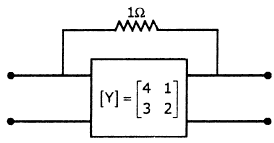Solution: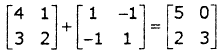There are two networks connected in parallel. Thus there Y-parameter will be added.

*Answer can only contain numeric values
QUESTION: 2

###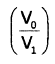in the circuit shown below is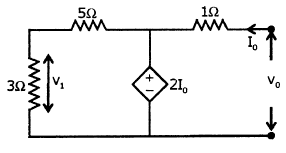Solution: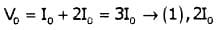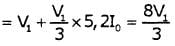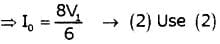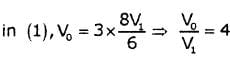QUESTION: 3

### The value of zin for the circuit shown below is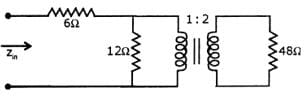Solution:

Net impedance on primary side of transformer is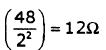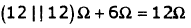*Answer can only contain numeric values
QUESTION: 4

Equivalent resistance across AB is _____Ω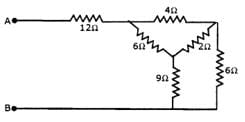Solution:

Convert Δ to Y and then use series parallel combination

QUESTION: 5

In the circuit given below v,n = u(—t) + u(t) V

Then vc (t) for t > 0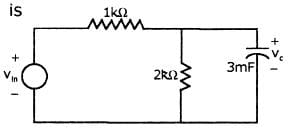Solution: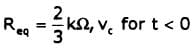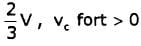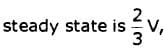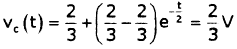QUESTION: 6

A voltage V = 12√2 cos 5000t V is applied to the circuit shown below.

The current i(t) is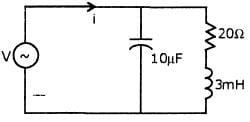Solution: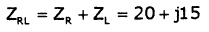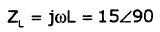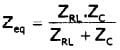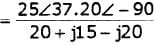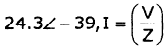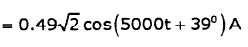QUESTION: 7

In the circuit shown below, the voltage source has been connected for a long time enough for steady state condition to be reached. At time t = 0, switch S is opened. Then open circuit voltage across AB is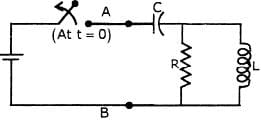Solution:
QUESTION: 8

Calculate Vx & Ix in the circuit shown below.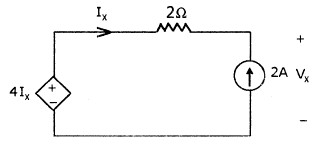Solution:

Ix = -2A
Apply KCL
-4Ix + 2Ix + Vx = 0
Vx = 4Ix -2Ix = 2Ix = -4V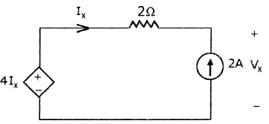QUESTION: 9

Determine the value of I' in the circuit shown below.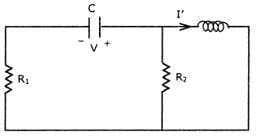Solution:
QUESTION: 10

Determine RMS values of voltage across capacitor (0.25F), current throughcapacitor, if voltage across capacitor is 4 + 4 cos (9t) - 5cos (4t)

Solution:

Vc = 4 + 4 cos(9t) - 5cos(4t)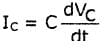= 1/4 {-4(9) sin(9t) + 5(4) sin (4t)}
⇒ -9 sin(9t) + 5 sin (4t)

RMS value of VL is =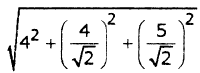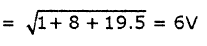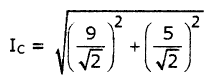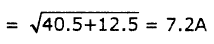*Answer can only contain numeric values
QUESTION: 11

The value of V, in the circuit shown below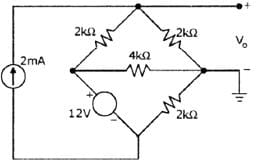Solution: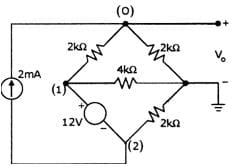V1— V2 =12V................. (1)

At node 0: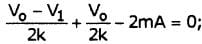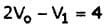--------(2)

At supernode 1 - 2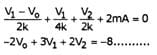(3)

Solving 1, 2 & 3; Vo = 4.5V; V1 = 5V; V2 = —7V

QUESTION: 12

For the circuit shown in figure, find V(t) when Vs = 2sin(500t) V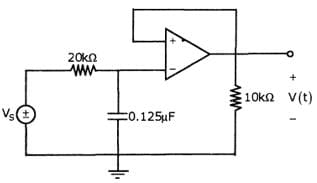Solution:
*Answer can only contain numeric values
QUESTION: 13

In the circuit shown below, the switch is closed at time t=0. The steady state value of the voltage Vc is_____ V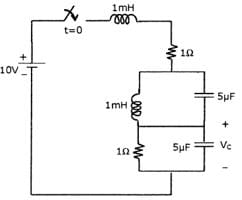Solution:

At steady state inductor short circuit, capacitor is open circuit. Thus voltage Vc is equal to voltage across 1 ohm resistance.

QUESTION: 14

Which of the following statements are true for the circuit shown below?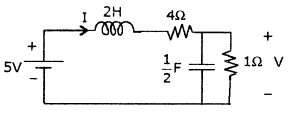1. It is first order with steady state value of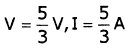2. It is second order with steady state value of  V = iV, I = 1A
3. The network function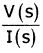has one pole I(s)
4. The network functionhas two poles I(s)

Choose the correct one

Solution: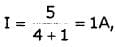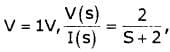Where V(t) is the voltage across the capacitor

This has a pole at S = -2.

QUESTION: 15

In the circuit shown below, it is found that the input ac voltage and current are in phase. The value of coupling coefficient K and the dot polarity of the coil PQ are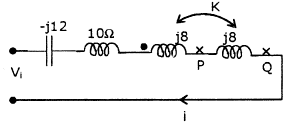Solution:

If input ac voltage and current are in phase then net impedance is equal to zero. Net inductive impedance = Net capacitive impedance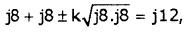, Thus k = 21 and dot must be at Q so that net inductance =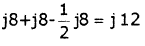QUESTION: 16

For the given circuit, switch closes at t = 0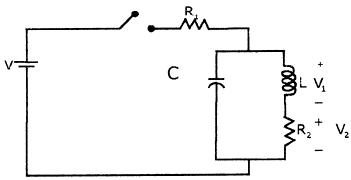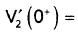Solution: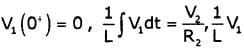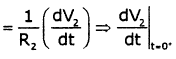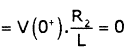QUESTION: 17

The voltage applied to a particular circuit comprising two components connected in series is given by:

V = (30 + 40 sin 103t +25sin2x103t +15sin4x103t) Vand the resulting current is given by

= 0.743 sin (103t + 1.190) + 0.781sin(2x103t + 0.896) + 0.636sin(4x103t + 0.559)A

Q. Average power supplied is

Solution:

Average Power P is given by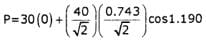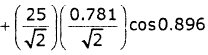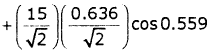P = 0 + 5.523+ 6.099+ 4.044 =15.67W

QUESTION: 18

The voltage applied to a particular circuit comprising two components connected in series is given by:

V = (30 + 40 sin103t +25sin2x103t +15sin4x103t) Vand the resulting current is given by

= 0.743 sin (103t + 1.190) + 0.781sin(2x103t + 0.896) + 0.636sin(4x103t + 0.559)A

Q. Which of the following element is not a part of the circuit?

Solution:

The expression for the voltage contains a dc component of 30V. However there is no corresponding term in the expression for current. This indicates that one of the components is a capacitor (since in a dc circuit a capacitor offers infinite impedance to a direct current). Since power is delivered to the circuit the other component is a resistor.

QUESTION: 19

Consider the circuit shown in figure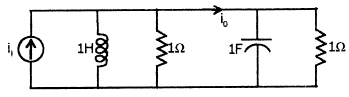Q.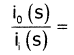Solution:

Using current division rule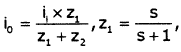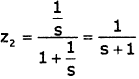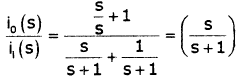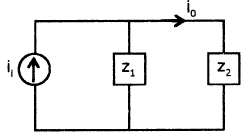QUESTION: 20

Consider the circuit shown in figureQ. If input current is δ(t), then io (t) (in Amps) will be

Solution: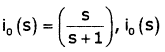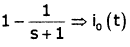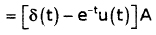Use Code STAYHOME200 and get INR 200 additional OFF Use Coupon Code